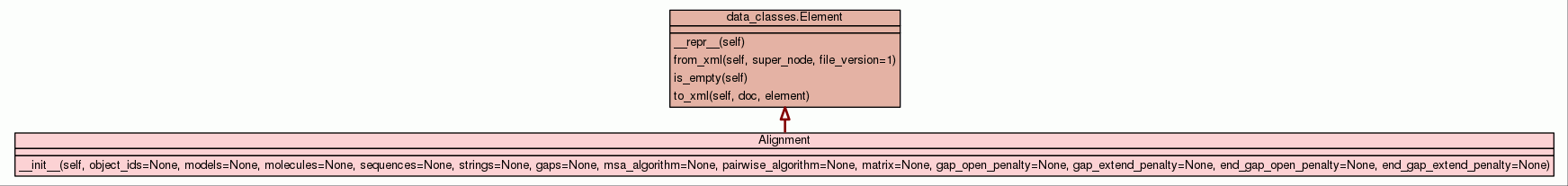[frames] | no frames]

# Class Alignment

source codeContainer for an individual sequence alignment.

 Instance Methods

 __init__(self, object_ids=None, models=None, molecules=None, sequences=None, strings=None, gaps=None, msa_algorithm=None, pairwise_algorithm=None, matrix=None, gap_open_penalty=None, gap_extend_penalty=None, end_gap_open_penalty=None, end_gap_extend_penalty=None) Set up the sequence alignment object. source code

Inherited from `data_classes.Element`: `__repr__`, `from_xml`, `is_empty`, `to_xml`

Inherited from `object`: `__delattr__`, `__format__`, `__getattribute__`, `__hash__`, `__new__`, `__reduce__`, `__reduce_ex__`, `__setattr__`, `__sizeof__`, `__str__`, `__subclasshook__`

 Properties

Inherited from `object`: `__class__`

 Method Details

### __init__(self, object_ids=None, models=None, molecules=None, sequences=None, strings=None, gaps=None, msa_algorithm=None, pairwise_algorithm=None, matrix=None, gap_open_penalty=None, gap_extend_penalty=None, end_gap_open_penalty=None, end_gap_extend_penalty=None)(Constructor)

source code

Set up the sequence alignment object.

Parameters:
• `object_ids` (list of str) - The list of IDs for each structural object in the alignment. In most cases this will be the data pipe name. This will be used to retrieve alignments.
• `models` (list of int) - The list of model numbers used in the alignment. This will be used to retrieve alignments.
• `molecules` (list of str) - The list of molecules used in the alignment. This will be used to retrieve alignments.
• `sequences` (list of str) - The list of residue sequences for the alignment as one letter codes.
• `strings` (list of str) - The list of alignment strings.
• `gaps` (numpy rank-2 int array) - The alignment gap matrix.
• `msa_algorithm` (str) - The global multiple sequence alignment (MSA) algorithm.
• `pairwise_algorithm` (str) - The pairwise sequence alignment algorithm.
• `matrix` (str) - The substitution matrix
• `gap_open_penalty` (float) - The penalty for introducing gaps, as a positive number.
• `gap_extend_penalty` (float) - The penalty for extending a gap, as a positive number.
• `end_gap_open_penalty` (float) - The optional penalty for opening a gap at the end of a sequence.
• `end_gap_extend_penalty` (float) - The optional penalty for extending a gap at the end of a sequence.
Overrides: object.__init__

 Generated by Epydoc 3.0.1 on Fri Jun 14 11:29:21 2019 http://epydoc.sourceforge.net